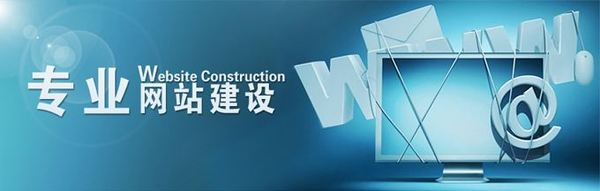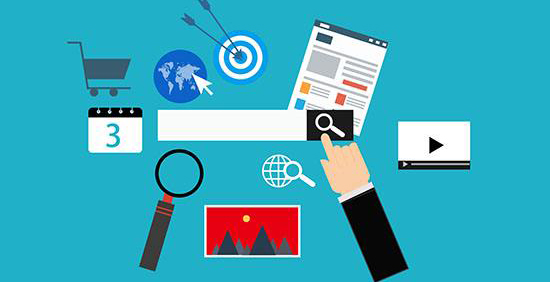免费咨询热线：023-6702-6306

# 利菲颖广告传媒——营销型企业网站！营销型企业是需要专职策划的，策划也不是盲目策划，不只是考虑网站的用色，内容的排版，还需要通过企业的服务，结合行业的痛点，从用户的需求出发，到消除客户的疑虑，从一个吸引用户，到用户喜欢，再到成交用户，都是需要策划来完善的;

细节是最能体验用户体验的，从一个按钮到一个页面的内容布局，都需要融合用户体验，在内容的丰富方面，能够有足够留得住用户的内容，在提升用户的时候，在推销产品，以用户为主，针对每个页面可指定跳转咨询广告文案、用户问答制定出一系列的整理方案，这样才能让营销型企业网站转化率得到有效提升;

营销型企业网站是满足搜索引擎优化的，能够很好的与搜索引擎之间建立互动，快速让蜘蛛抓取网站内部信息，很好的处理了用于优化的标签、动态页、内链等等，并且每一个页面都能独立设置关键词，能够让更多的长尾关键词获取排名，获取更多的精准流量，挖掘更多的潜在客户;总结，用户喜欢你这个网站就是因为体验做的好，内容有价值，营销型企业网站就是抓住了用户这一点，将营销的思想、方法和技巧融入到网站策划、设计与制作中的网站，在满足用户需求中，销售产品，所以这样的有营销力的网站，转化率是特别高的，也是符合企业现代的网络营销需求的，同时解决用户的需求。

<<<<<<<<<<<<<<<<<<<<<<<<<<<<<<<<<<<<<<<<<<<<<<<<<<<<<<<<<<<<<<<<<<<<<<<<<<<<<<<<<<<<